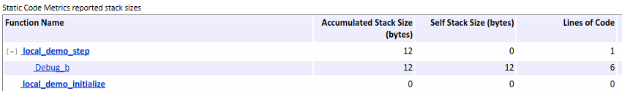## Static Code Metrics

### Static Code Metrics

The code generator performs static analysis of the generated C or C++ code and provides these metrics in the Static Code Metrics Report section of the HTML Code Generation Report. When you place your cursor over a function or a variable in the generated code, you can also see metrics.

You can use the information in the report to:

• Find the number of files and lines of code in each file.

• Estimate the number of lines of code and stack usage per function.

• Compare the difference in terms of how many files, functions, variables, and lines of code are generated every time you change the model or MATLAB® algorithm.

• Determine a target platform and allocation of RAM to the stack, based on the size of global variables plus the estimated stack size.

• Determine possible performance slow points, such as the largest global variables or the most costly call path in terms of stack usage.

• View the cyclomatic complexity of a function, which counts the number of linearly independent paths through a function.

• View the function call tree. Determine the longest call path to estimate the worst case execution timing.

• View how target functions, provided by the selected code replacement library, are used in the generated code.

For examples, see:

### Static Code Metrics Analysis

Static analysis of the generated code is performed only on the source code without executing the program. The results of the static code metrics analysis are included in the Static Code Metrics section of the HTML Code Generation Report. The report is not available if you generate a MEX function from MATLAB code.

Static analysis of the generated source code files:

• Uses the specified C data types. For Simulink® models, you specify these data types in the Hardware Implementation > Production hardware pane of the Configuration Parameters dialog box. For code generation from MATLAB code, you specify them in the Hardware tab of the MATLAB Coder™ project settings dialog box or using a code generation configuration object. Actual object code metrics might differ due to target-specific compiler and platform settings.

• Includes custom code only if you specify it. For Simulink models, you specify custom code on the Code Generation > Custom Code pane in the model configuration. For code generation from MATLAB code, you specify it on the Debugging tab of the MATLAB Coder project settings dialog box or using a code generation configuration object. An error report is generated if the generated code includes platform-specific files not contained in the standard C run-time library.

• For Simulink models, includes the generated code from referenced models.

• Uses 1-byte alignment for all members of a structure for estimating global and local data structure sizes. The size of a structure is calculated by summing the sizes of all of its fields. This estimation represents the smallest possible size for a structure.

• Calculates the self stack size of a function as the size of local data within a function, excluding input arguments. The accumulated stack size of a function is the self stack size plus the maximum of the accumulated stack sizes of its called functions. For example, if the accumulated stacks sizes for the called functions are represented as `accum_size1...accum_sizeN`, then the accumulated stack size for a function is

`accumulated_stack_size = self_stack_size + max(accum_size1,...,accum_sizeN)`

For example consider this piece of generated code:Now consider the code metrics shown below:The accumulated stack size is 12 bytes because the self stack size of the function `Debug_b` is 12 bytes and the 4 input arguments do not contribute to the calculation of accumulated stack size.

• When estimating the stack size of a function, static analysis stops at the first instance of a recursive call. The Function Information table indicates when recursion occurs in a function call path. Code generation generates only recursive code for Stateflow event broadcasting and for graphical functions if it is written as a recursive function.

• Calculates the cyclomatic complexity of a function as the number of decisions plus one:

`CC = Number of decisions + 1`
The following constructs add a decision:

• If statement

• Else-If statement

• Switch statement (1 decision for each `case` branch)

• Loop statements: While, For, Do-while

Note

Boolean operators in the preceding constructs do not add extra decisions.

• Does not include `ert_main.c`, because you have the option to provide your own `main.c`.

### View Static Code Metrics and Definitions Within the Generated Code

When you view code in the Code view or in the code generation report, place your cursor over the ellipsis menu above global variables and functions to see code metrics information.The tooltip provides a link to the definition for the variable or function.### Static Code Metrics Report Limitations

Static code metrics are not available if the target configuration for a model results in generated code that:

• Includes a header file that is not generated by the model hierarchy and is not a system header file

• Uses a macro that is not recognized